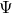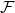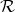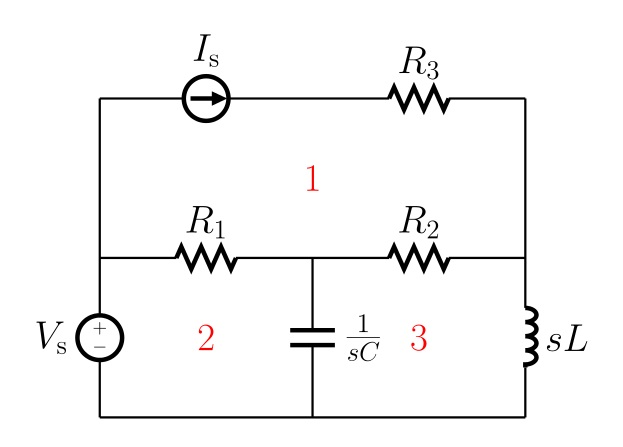Hi again, and welcome back to the fascinating world of reluctance networks! After last post‘s brief detour to the grim world of how-not-to, let’s get back to more positive matters. In other works, let’s see how solving reluctance networks happens in practice.

As should be clear from the previous posts in the series, reluctance networks are quite similar indeed to electric networks. Thus, similar approaches can be used to analyse them.

Obviously, we have to replace currents by the fluxes, and voltage drops over resistances by mmf dropsover reluctances. Where things can differ more, are the mmf sources from the currents in the problem domain. More about that – again – very shortly.

But let’s move on to the solution methods.

### Simple, yet limited

Most people seem to use the nodal method, due to its simplicity. In the nodal method, each point where two or more reluctances are connected to each other is called a node. Then, with some simple mathematics, a (reduced) magnetic scalar potential is solved for each node. Despite the fancy name, the potential pretty much corresponds to the more-well-known electric potential.

Indeed, the mmf difference over any reluctance is equal to the magnetic potential difference between its end-nodes. And from this drop, the flux going through it is easily obtained. You see, exactly like when using the nodal method for analysing electric circuits.

The method is very easy to use, both by hand and programmatically alike. Indeed, we only need to know if two nodes are connected by a reluctance or not.

### Complex, but general

However, there’s a fundamental limitation in the method. As I ranted last time, magnetic fields are typically rotational, while the electric fields found in typical circuits are not. Thus, taking the currents properly into account, by only using simple “magnetic voltage” elements in series with the reluctances, is not a trivial problem. It can probably be done, but it’s not easy.

The simplest solution to the problem, in my opinion, is simply not do it. Can’t be much simpler than that, right?

Instead, let’s use the loop method and adopt a set of virtual loop fluxes as the unknowns. Exactly like we would do in electric circuit analysis, only now with fluxes instead of currents. You still with me?Illustration of the loop method for electric circuit, because figures are nice. Source and license here.

This way, we don’t have to worry about how to transform the current-mmfs into simple circuit elements. Instead, we simply compute the total current enclosed by a flux loop (using surface integral in the general case, or any point-in-polygon method in 2D problems), and simply call that the source term for that current loop.

Simple as that.

#### But, how?

Unfortunately, forming the flux loops is not as simple as that. For simple networks, it can be done by hand. For more complex ones – not so much.

And of course, doing things by hand does not lend itself well to fully-automatic programmatic analysis.

Luckily, an algorithmic solution exists for this problem, called the fundamental loop method.

We begin – again – by calling each junction of two or more reluctance elements a node. Then, we form a spanning tree for the nodes, of the reluctance elements. This is easy to do with any Computer Science 101 -style algorithm of your choice.

Now, since the spanning tree is a spanning tree, each of the nodes will be in it, but the reluctances will not form any loops at all.

Next, we take one reluctance element that is not in the tree. Obviously, it’s end-nodes can be found in the tree. Next thing, we find a path inside the tree that connects these nodes to each other. Since there are no loops in the tree, this path is unique and easy to find, algorithmically.

Finally, we combine the single reluctance element outside the tree, and all the elements lying on the path inside the tree, into a continuous loop. This loop, painfully obviously, is our first flux loop.

Then, we just repeat this procedure for each reluctance element outside the tree, and obtain a flux loop associated with each.

And some wise old men – or maybe women, not sure – have proven that this set of loops is sufficient to uniquely express all the actual fluxes flowing in the reluctance elements of the network.

I’d call that a victory.

That’s all for today. In the future, we’ll talk about some details of the loop method and non-linear analysis. But first, let’s consider a practical example!

Need help with electric motor design or design software? Let's get in touch - satisfaction guaranteed!RN 103: Solving reluctance networks